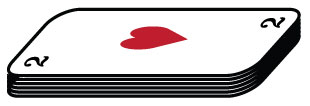# Magical CardsA deck of $2n$ cards is numbered $1, 2, \cdots, 2n$ from top to bottom. The top $n$ cards are removed, kept in order, and put into another pile A. The remaining cards in the original deck are in pile B. Then, the top card is taken from pile B and placed on the table, then the top card from pile A is placed on top of that, then the new top card on pile B is placed on top of that, and so forth until all cards are exhausted. Define a card position $m$ to be magical if there exists a deck of cards such that the card in position $m$ retains its original position after the process. As an explicit example, $3$ and $6$ are magical because in a deck of $8$ cards, $3$ and $6$ are still in the $3rd$ and $6th$ positions, respectively, counting from the top after the process.

How many integers $m < 1000$ are not magical?

Details and Assumptions

A deck of cards must have an even number of cards by definition.

×